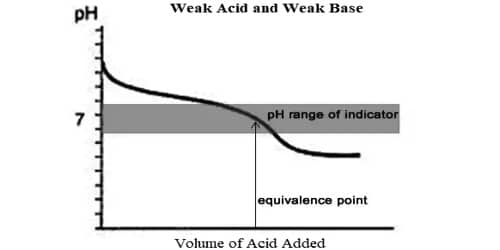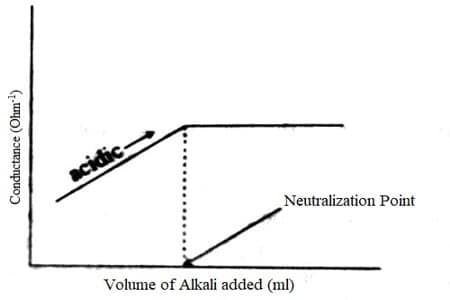Chemistry

# Conductometric Titration of Weak Acid and Weak BaseConductometric Titration of a weak acid and weak base:

The conductance of electrolytes depends on the number of ions and their speeds. For conductometric titration experiments, a known volume of the solution to be titrated is placed in a beaker and a conductivity cell dipped into it. The conductivity cell is now connected to one end of the Wheatstone’s bridge.

The principle of conductometric titration is based on the detail that throughout the titration, one of the ions is replaced by the other and consistently these two ions diverge in the ionic conductivity with the consequence that conductivity of the solution varies throughout the course of titration.

One major feature of weak acids and bases is that they do not dissociate completely in water, producing less free oxonium or hydroxide ions than strong acids and bases do. If both are of equal strength, then the equivalence pH will be neutral.

For weak acid and weak base the conductance initially rises due to the formation of a salt, say ammonium ethanoate from Ammonium hydroxide (NH4OH) and Acetic acid (CH3COOH). On further addition of NH4OH, the conductance continually raises till the neutral point is reached.

After this, the conductance remains almost constant because NH4OH is a very weak base and contributes only very slightly to conductance. The titration cane for a weak acid – weak base is shown in Figure. The point of intersection of the two linear portions gives the neutral point. Almost all acid-base titrations can be performed conductometrically.Fig: Titration of weak acid vs weak base

Similarly, if you titrate sodium hydroxide solution with ethanoic acid, at the equivalence point the pure sodium ethanoate formed has a slightly alkaline pH because the ethanoate ion is slightly basic. For example in Sodiumacetate, a weak base, there is an equilibrium where the reactions left to right and right to left occur at the same speed, hence canceling each other.

The equivalence point may be situated graphically by plotting the transform in conductance as a function of the volume of titrant added. After the equivalence point, conductance virtually remains similar to the weak base which is being added is feebly ionized and, therefore, is not much conducting. The endpoint for this titration research occurs at the intersection of the two straight lines in the graph.Remember the following features:

• The curve resembles the weak acid – strong base conductance curve in the previous section up to the endpoint for the titration.
• Past the equivalence point for the neutralization reaction, the addition of more weak base [NH4OH(aq)] (Ammonium hydroxide) has little impact on the conductance of the solution since its dissociation is depressed by the presence of ammonium ions, NH4+(aq), already nearby in the solution.
• The endpoint for this weak acid – weak base titration experiment is much easier to notice than for the weak acid – strong base titration experiment.
• We can determine the volume of weak base needed to neutralize all the weak acid at the equivalence point for the neutralization reaction by reading it straight off the graph.